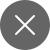# Understanding XG Boost- A Basic Overview

7+

## What led to the need for Boosting technology?

Let’s know the need for Boosting in machine learning through an example.

Example: We have to recognize two objects as cat and dog, and we will identify them with the help of few rules like if the image has point ears then it is a cat, if the image has bigger limbs then it is a dog, if the image has wider mouth then again it is a dog and if the image has sharp claws then it is a cat. These are some we define to recognize the animal.

Using only a single rule we cannot define whether the animal is a cat or a dog. Each of these rules individually is weak learners that are not accurate in identifying the images. So, to get more accurate predictions we will be combining our predictions from each of these weak learners by using the majority rule. This majority rule gives us strong learners.

## What is Boosting?

Boosting is an ensemble learning technique that uses a set of Machine learning algorithms to combine weak learners and form a strong learner to increase the accuracy of the model. It improves the prediction accuracy of the model.

What boosting does is that it generates multiple weak learners and combine their predictions to form one strong rule. These weak learners are generated by applying base machine learning algorithms on different distributions of the data set. By default, these base learning algorithms are decision trees in boosting.

Types of Boosting algorithms:

• XGBoost Algorithm

## Ensemble Learning

Ensemble learning improves the results of machine learning algorithms by combining models to produce more accurate results. It gives us a systematic solution by combining the predictive results of multiple learners.

## XGBoost

XGBoost is a library and it abbreviates to Extreme Gradient Boosting. XGBoost was introduced in 2014. It is the implementation of gradient boosting decision trees that increase the speed of the model and performance. It uses a gradient framework, and it is an ensemble learning because we cannot rely upon a single model for accurate results.

Artificial neural networks perform well when the data is unstructured but, when the data is small and structured, we use decision trees for prediction. This is the reason why we use decision tree-based algorithms in XGBoost. We use XGBoost commonly in supervised learning in Machine learning.

XGBoost is the software library that we can download and run in our own systems. It supports the following properties:

• XGBoost supports programming languages like c++, python, R, Java, Julia, and platforms like Hadoop
• XGBoost has a wide range of applications. We use XGBoost to solve Classification, Regression, Ranking, and user-defined problems.
• We can run XGBoost on Windows, macOS, Linux, etc.

## Working of XGBoost

Boosting is nothing more than an ensemble technique where we resolve the errors of the previous model in the new model. We add up these models until there is no difference between the previous and current models.

Gradient boosting is a technique that creates new models that compute the errors of the previous model and we add the leftover part to get the final prediction.

The main advantages of the XGBoost are:

• Execution Speed: XGBoost execution is quite faster than the remaining implementations of gradient boosting.
• Model performance: XGBoost is an ensemble tree method that uses the principle of boosting weak learners with the help of gradient decent architecture. This increases the accuracy of the model.

Let’s see the reason behind the good performance of XGBoost.

• Cross-Validation: In general, we import the cross-validation function from sklearn but XGBoost consists of a cross-validation function inbuilt.
• Regularization: This is the most crucial and dominant factor of the model. Regularization is the technique through which we can get rid of the overfitting of the model.
• Missing Value: XGBoost is designed in such a way that it handles the missing values. This finds out the missing values and includes them off-late.
• Flexibility: XGBoost supports objective functions. These objective functions are the functions that we use to evaluate the performance of the model. This also handles the validation of user-defined metrics.
• Save and Load: It will give the power to save the data and reloads the data whenever required.

## Conclusion

In this article, I have given an introduction to the XGBoost library. Machine learning is a very wide area and there are many chances to XGBoost. It increases execution speed and performance.7+

### 2 Responses

1.Pon Pradeep D says: